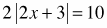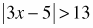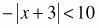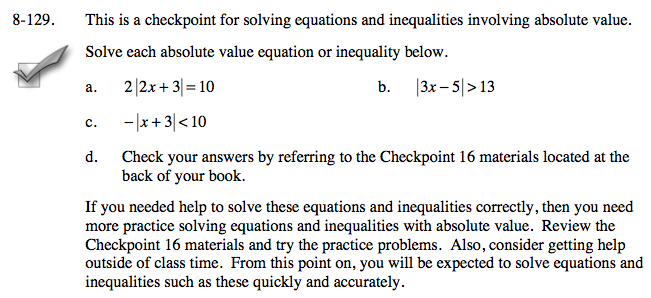### Home > A2C > Chapter 8 > Lesson 8.2.2 > Problem8-129

8-129.
1. This is a checkpoint for solving equations and inequalities involving absolute value.Solve each absolute value equation or inequality below.

1.2.3.4. Check your answers by referring to the Checkpoint 16 materials located at the back of your book.

If you needed help to solve these equations and inequalities correctly, then you need more practice solving equations and inequalities with absolute value. Review the Checkpoint 16 materials and try the practice problems. Also, consider getting help outside of class time. From this point on, you will be expected to solve equations and inequalities such as these quickly and accurately. Homework Help ✎Answers and extra practice are located in the back of your printed textbook or in the Reference Tab of your eBook.
If you have an eBook for A2C , login and then click the following link: Checkpoint 16 Answers and Practice